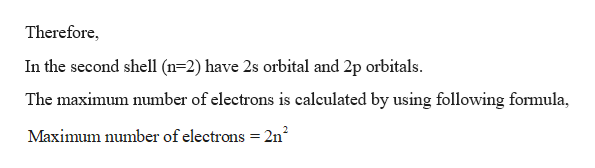# What is the maximum number of electrons in an n = 2 shell?

Question

What is the maximum number of electrons in an n = 2 shell?

check_circleExpert Solution
Step 1

Orbital:

Orbital, a mathematical expression, called a wave function, an orbital is used to find the electron.

Number of electrons in the shells is given below,

Each shell contains only a fixed number of electrons. T...help_outlineImage TranscriptioncloseTherefore In the second shell (n 2) have 2s orbital and 2p orbitals The maximum number of electrons is caleulated by using following formula, Maximum number of electrons 2n2 fullscreen

### Want to see the full answer?

See Solution

#### Want to see this answer and more?

Solutions are written by subject experts who are available 24/7. Questions are typically answered within 1 hour*

See Solution
*Response times may vary by subject and question
Tagged in

### General Chemistry